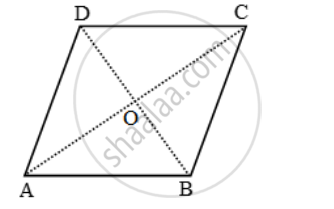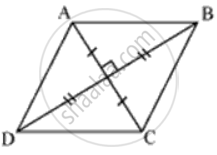# ABCD is a rhombus. Prove that AB^2 + BC^2 + CD^2 + DA^2= AC^2 + BD^2 - Mathematics

Sum

ABCD is a rhombus. Prove that AB2 + BC2 + CD2 + DA2= AC2 + BD2

#### Solution 1

Let the diagonals AC and BD of rhombus ABCD intersect at O.

Since the diagonals of a rhombus bisect each other at right angles.

∴ ∠AOB = ∠BOC = ∠COD = ∠DOA = 90º and AO = CO, BO = OD.

Since ∆AOB is a right triangle right-angle at O.∴ AB2 = OA2 + OB2

AB^2=(1/2AC)^2 +(1/2BD)^2

⇒ 4AB2 = AC2 + BD2 ….(i)

Similarly, we have

4BC^2 = AC^2 + BD^2 ….(ii)

4CD^2 = AC^2 + BD^2 ….(iii)

and,

4AD^2 = AC^2 + BD^2 ….(iv)

Adding all these results, we get

4(AB^2 + BC^2 + AD^2 ) = 4(AC^2 + BD^2 )

⇒ AB^2 + BC^2 + AD^2 + DA^2 = AC^2 + BD^2

#### Solution 2In ΔAOB, ΔBOC, ΔCOD, ΔAOD
Applying Pythagoras theroem
BC2 = BO2 + OC2
CD2 = CO2 + OD2
AB2 + BC2 + CD2 + AD2
= 2(AD2 + OB2 + OC2 + OD2)

= 2(("AC"/2)^2 + ("BD"/2)^2 + ("AC"/2)^2 + ("BD"/2)^2)  ...(diagonals bisect each othar.)

= 2(("AC")^2/2 + ("BD")^2/2)
= (AC)2 + (BD)2.

Concept: Right-angled Triangles and Pythagoras Property
Is there an error in this question or solution?

#### APPEARS IN

Frank Class 9 Maths ICSE
Chapter 17 Pythagoras Theorem
Exercise 17.1 | Q 17